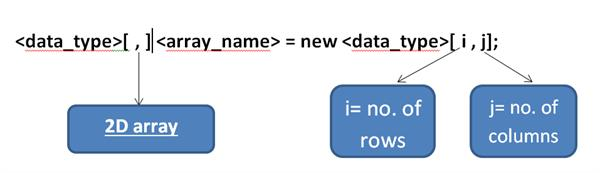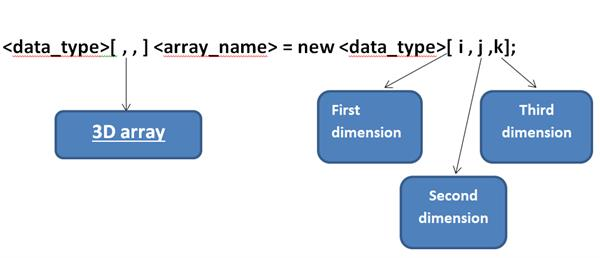Loading, please wait...# What are the Arrays in C# | C# Arrays in Details

This article helps in understanding the various types of arrays in C# programming language.

## Arrays In C# with examples

Arrays are the storage elements used to store multiple values under a single variable name having the same data type.

Consider a case in which we want to store 10 integer values in a program. Instead of declaring 10 different variables, we can simply use an array having a single variable name and declare its size to be 10.

DECLARATION:

An array is declared in a below-given way:

[ ]

For example:

To declare an array of integer type with name “arr” and size 10, we will have to declare in the following manner:

``Int[ ] arr = new int;``

This statement will allocate a contiguous memory location having 10 blocks, where each block will hold an integer value ‘0’ (zero) by default until an integer value is assigned to that particular block.

Note: It is to be noted that the position of the array blocks is termed as its index value and it always starts with index 0.

There are three types of arrays in C# :

1. One-dimensional array
2. Multi-dimensional array
3. Jagged array

These are described below:

### One-dimensional array in C#:

In a one-dimensional array, the elements are stored in a linear fashion.

These elements can be accessed by specifying the position of every array element in single index value.

Declaration:

A 1-D array is declared as follows:

string [ ] ar1 = new string;

Where ar1 is the name of an array that can hold 5 string values in the array.

The values can be assigned as :

``````string [ ] ar1 = new string {“red”,”blue”,”green”,”purple”,”orange”};

or

string [ ] ar1 = new string;

ar1 = “red”;

ar1= “blue”;

ar1= “green”;

ar1= “purple”;

ar1= “orange”;``````

A program to illustrate one-dimensional array is given here:

``````using System;

namespace OneDim
{
class Program
{
static void Main(string[] args)
{
int[] ar = new int;
Console.WriteLine("enter digits");
for(int i=0; i<4; i++)
{
}
int sum = 0;
for (int i = 0; i < 4; i++)
{
sum = sum + ar[i];
}
Console.WriteLine("\n The sum is");
Console.WriteLine(sum);
}
}
}``````

Explanation: This program will ask the user to enter four integer values and then calculate and print their sum.

### Multi-dimensional array in C#:

In multi-dimensional arrays, the data or the array elements are stored in a tabular form or matrix form.

These arrays are also called rectangular arrays due to the presence of rows and columns making it 2D or 3D.

Declaration:

A multidimensional array is declared by using commas within the square brackets.

A 2D array uses a single comma and the 3D array consists of double commas. This will be more clear by the below-given example:### Declaration of a 2D array### Declaration of a 3D array

#### Assigning values in a multidimensional array:

Values in a multidimensional array are assigned as follows:

``````int [ , ] arr1 = new int [2 ,3] {{1,2,3} , {1, 2, 3}};

or

int [ , ] arr1 = new int [2,3];

arr1[0,0] = 1;

arr1[0,1]= 2;

arr1[0,2] = 3;

arr1[1,0]= 1;

arr1[1 ,1]= 2;

arr1[1, 2]= 3;``````

A program to illustrate a multi-dimensional array is given below:

``````using System;

namespace multi
{
class Program
{
static void Main(string[] args)
{
int[,] ar = new int[2, 2];
int[,] ar1 = new int[2, 2];
int[,] ar2 = new int[2, 2];

int i, j;
Console.WriteLine("\n ENTER FIRST MATRIX");
for (i = 0; i < 2; i++)
{
for (j = 0; j < 2; j++)
{
}
}
for (i = 0; i < 2; i++)
{
for (j = 0; j < 2; j++)
{
Console.Write(ar[i, j]+"\t \t \t \t \t");
}
Console.WriteLine("\n");
}
Console.WriteLine("\n ENTER SECOND MATRIX");
for (i = 0; i < 2; i++)
{
for (j = 0; j < 2; j++)
{
}
}
for (i = 0; i < 2; i++)
{
for (j = 0; j < 2; j++)
{
Console.Write(ar1[i, j] + "\t \t \t \t \t");
}
Console.WriteLine("\n");
}
for (i = 0; i < 2; i++)
{
for (j = 0; j < 2; j++)
{
ar2[i, j] = ar1[i, j] + ar[i, j];
}
}
Console.WriteLine("sum is" + "\n");
for (i = 0; i < 2; i++)
{
for (j = 0; j < 2; j++)
{
Console.Write(ar2[i, j] + "\t \t \t \t \t");
}
Console.WriteLine("\n");
}
}
}
}``````

EXPLANATION: This program will ask the user to enter values in two 2D matrices and then calculate and print the sum of the matrices in a third matrix.

## Jagged Array in C#

A jagged array is a collection of arrays or we can say an array of arrays is called a jagged array.

The arrays present in the jagged array can be of any size or length.

In other words, the jagged arrays store arrays instead of actual values.

The members of a jagged array are of reference types and these are initialized as null by default.

Declaration:

• A jagged array is declared by using two square brackets.
• The first bracket specifies the number of separate arrays stored in the jagged array.
• The second bracket tells about the type of arrays stored in the jagged array. i.e, whether a single-dimensional array or multi-dimensional array.

Example:

int[ ][ ] arr1 = new int [  ];

• This will declare a jagged array containing two single-dimensional arrays.

int[ ][ ] arr1 = new int [ , ];

• This will declare a jagged array containing four multi-dimensional arrays.

Initialization:

``````int[ ][ ] arr1 = new int [  ];

arr1 = new int{1, 2, 3, 4};

arr1 = new int {1, 3};

or

int[ ][ ] arr1 = new int [  ] {new int{1, 2, 3, 4}, new int {1, 3}};``````

A program to illustrate the use of a jagged array is given below:

``````using System;
namespace jagged
{
class Program
{
static void Main(string[] args)
{
int[][] arr1 = new int[] { new int { 10, 20, 30, 40 }, new int { 100, 200 } };
for(int i=0; i``````
``` ```
``` ```
``` ```
``` Related Videos C# Program For Check Total Occurrence Of A Number In An Array Program To Check Whether A Number Is A Perfect Number Or Not Structs and Enums in C# | C# Tutorials Inheritance in C# | C# Tutorials Operator overloading and Indexers in C# | C# Tutorial The Static keyword in C# | C# Tutorial C# Constructor and Properties | C# Tutorials What are Arrays in C# | C# Tutorials ```
``` \$(function () { //\$("#topleaderboard").load("/Home/Ads_load?placeid=2"); \$("#authorblock").load("/Home/Article_Author?id=1427"); \$("#divads1").load("/Home/Ads_load?placeid=6"); \$("#divads2").load("/Home/Ads_load?placeid=7"); }) ```
```COMPANY About Us Contact Us Privacy Policy Become An Author JOIN TUTORIALS LINK Our Newsletter Will Let You Know When Any NewArticles, Tutorials and Video Are Released. CONTRIBUTE Article News Video Ebook Code Snippet MOBILE APPGoogle Play Follow us About Us Contact Us Privacy Policy Become An Author Student Login Services Copyright © 2021. All Rights Reserved. ```
``` \$(function () { \$("#divLoading").hide(); }) \$(function () { if (screen.width <= 700) { landscapeMode(); } }) ```